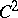# Theory of surfaces

The branch of differential geometry dealing with surfaces. In the theory of surfaces one examines the shape of a surface, its curvature, the properties of various types of curves on a surface, aspects of deformation, the existence of a surface with given internal or external features, etc.

There are two points of view in the theory of surfaces: A surface can be considered as a metric space with its own intrinsic metric (so-called intrinsic geometry) or as a figure in space (so-called extrinsic geometry). Many trends in the theory of surfaces (questions of isometric immersion, isometric deformation, cf. Deformation, isometric, etc.) deal with the links between intrinsic and extrinsic geometry. The links are fairly close for regular surfaces of class. See also Geometry of immersed manifolds.

The study of the behaviour of curves on surfaces (asymptotic lines, geodesic lines, etc., cf. Asymptotic line; Geodesic line) and pairs of one-parameter families of them (so-called nets, cf. Net (in differential geometry)) has led to the distinction of special classes of surfaces. For example, a translation surface is characterized by the existence of a transport net on it, a Voss surface is characterized by a Voss net, a ruled surface has a semi-geodesic asymptotic net, etc. The theory of nets is closely related to aspects of mapping surfaces onto surfaces. The following are the most important classes of mappings: isometric mappings (cf. Isometric mapping), which preserve arc lengths, areas and angles between two directions emanating from a point; conformal mappings (cf. Conformal mapping), which preserve angles between any two directions emanating from one point (for example, the stereographic projection); spherical mappings (cf. Spherical map), under which each point on a surface is put into correspondence with the point on the sphere under parallel transfer of normals; geodesic mappings (cf. Geodesic mapping), under which the geodesics of one surface correspond to the geodesics of the other; and, finally, equi-areal mappings, under which the areas of corresponding figures are in constant ratio.

Studies in the theory of surfaces involve examining various classes of surfaces, such as surfaces of the second order, surfaces of screw motion, helicoids, Catalan surfaces, conoids, regression surfaces, canal surfaces, Dupin cyclides, Enneper surfaces, Weingarten surfaces, etc. (cf. Canal surface; Catalan surface; Conoid; Dupin cyclide; Enneper surface; Helicoid; Regression surface; Surface of the second order; Surface of screw motion; Weingarten surface). Much attention has been given to minimal surfaces (cf. Minimal surface). In relation to applications, much attention has been given to triorthogonal systems of surfaces (cf. Triorthogonal system of surfaces), e.g. confocal second-order surfaces.

In addition to the properties of surfaces that are unaltered under isometric transformation of the entire space (which relate to the so-called metric theory of surfaces), studies have been made on the properties of surfaces that are invariant under some other group of transformations, for example, the groups of affine or projective transformations. The affine theory of surfaces considers the properties of surfaces that are unaltered under equi-affine transformations (i.e. affine transformations that preserve the volume). The projective theory of surfaces deals with projectively-invariant properties. See also Affine differential geometry; Conformal-differential geometry; Projective differential geometry.

Studies on the theory of surfaces, and studies on fields of geometric objects associated with surfaces, have stimulated the development of many methods that have found an application in other branches of mathematics, physics and mechanics (for example, tensor analysis, the Cartan method of exterior forms, etc.).

The theory of surfaces in the form it took at the end of the 19th century dealt only with surfaces (or parts of it) that are reasonably regular for the use of differential calculus. At the beginning of the 20th century, lines of research developed in which the requirements of differentiability were not imposed, this being balanced by certain other requirements to ensure that one obtained significant geometrical results. Such requirements are convexity (see Convex surface) and local uniqueness of geodesics. Also, the studies were extended to surfaces in their full extent (so-called geometry in the large), and not to fairly small parts, as was the case in classical differential geometry. Here it was important to incorporate the topological structure (for example, the genus of a surface), the completeness (in some sense) and the relationship between these characteristics and the curvature distribution.

Studies on the differential geometry of multi-dimensional spaces, elliptic spaces and Lobachevskii spaces have established various geometrical facts relating to the theory of surfaces in these spaces.